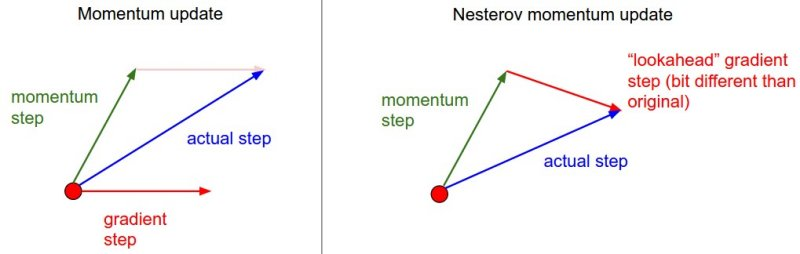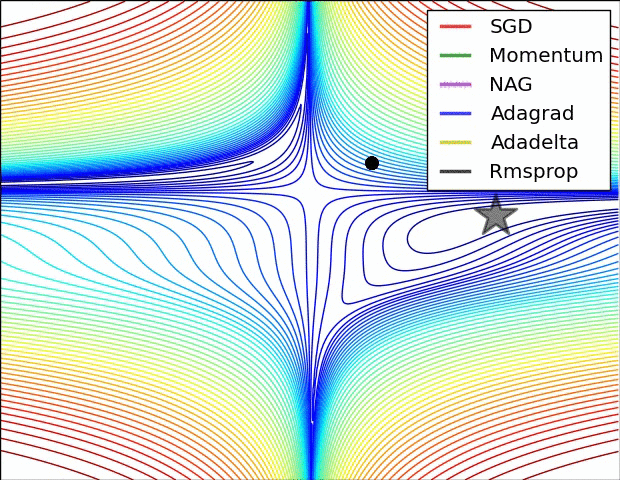# 1. SGD

### Batch Gradient Descent

$\Theta = \Theta -\alpha \cdot \triangledown_\Theta J(\Theta )$

• cost fuction若为凸函数，能够保证收敛到全局最优值；若为非凸函数，能够收敛到局部最优值

• 由于每轮迭代都需要在整个数据集上计算一次，所以批量梯度下降可能非常慢
• 训练数较多时，需要较大内存
• 批量梯度下降不允许在线更新模型，例如新增实例。

### Stochastic Gradient Descent

$\Theta = \Theta -\alpha \cdot \triangledown_\Theta J(\Theta ;x^{(i)},y^{(i)})$

• 算法收敛速度快(在Batch Gradient Descent算法中, 每轮会计算很多相似样本的梯度, 这部分是冗余的)
• 可以在线更新
• 有几率跳出一个比较差的局部最优而收敛到一个更好的局部最优甚至是全局最优

• 容易收敛到局部最优，并且容易被困在鞍点

### Mini-batch Gradient Descent

mini-batch Gradient Descent的方法是在上述两个方法中取折衷, 每次从所有训练数据中取一个子集（mini-batch） 用于计算梯度：
$\Theta = \Theta -\alpha \cdot \triangledown_\Theta J(\Theta ;x^{(i:i+n)},y^{(i:i+n)})$

• 选择适当的学习率$\alpha$ 较为困难。太小的学习率会导致收敛缓慢，而学习速度太块会造成较大波动，妨碍收敛。
• 目前可采用的方法是在训练过程中调整学习率大小，例如模拟退火算法：预先定义一个迭代次数m，每执行完m次训练便减小学习率，或者当cost function的值低于一个阈值时减小学习率。然而迭代次数和阈值必须事先定义，因此无法适应数据集的特点。
• 上述方法中, 每个参数的 learning rate 都是相同的，这种做法是不合理的：如果训练数据是稀疏的，并且不同特征的出现频率差异较大，那么比较合理的做法是对于出现频率低的特征设置较大的学习速率，对于出现频率较大的特征数据设置较小的学习速率。
• 近期的的研究表明，深层神经网络之所以比较难训练，并不是因为容易进入local minimum。相反，由于网络结构非常复杂，在绝大多数情况下即使是 local minimum 也可以得到非常好的结果。而之所以难训练是因为学习过程容易陷入到马鞍面中，即在坡面上，一部分点是上升的，一部分点是下降的。而这种情况比较容易出现在平坦区域，在这种区域中，所有方向的梯度值都几乎是 0。

# 2. Momentum

SGD方法的一个缺点是其更新方向完全依赖于当前batch计算出的梯度，因而十分不稳定。Momentum算法借用了物理中的动量概念，它模拟的是物体运动时的惯性，即更新的时候在一定程度上保留之前更新的方向，同时利用当前batch的梯度微调最终的更新方向。这样一来，可以在一定程度上增加稳定性，从而学习地更快，并且还有一定摆脱局部最优的能力：

$v_{t} = \gamma \cdot v_{t-1} + \alpha \cdot \triangledown_\Theta J(\Theta )$
$\Theta = \Theta-v_{t}$

Momentum算法会观察历史梯度$v_{t-1}$，若当前梯度的方向与历史梯度一致（表明当前样本不太可能为异常点），则会增强这个方向的梯度，若当前梯度与历史梯方向不一致，则梯度会衰减。**一种形象的解释是：**我们把一个球推下山，球在下坡时积聚动量，在途中变得越来越快，γ可视为空气阻力，若球的方向发生变化，则动量会衰减。

# 3. Nesterov Momentum

$v_{t} = \gamma \cdot v_{t-1} + \alpha \cdot \triangledown_\Theta J(\Theta -\gamma v_{t-1})$
$\Theta = \Theta-v_{t}$$\Theta_{t+1,i} =\Theta_{t,i}-\alpha \cdot g_{t,i}$

Adagrad在每轮训练中对每个参数$θ_i$的学习率进行更新，参数更新公式如下：

$\Theta_{t+1,i} =\Theta_{t,i}- \frac{\alpha}{\sqrt{G_{t,ii}+\epsilon }}\cdot g_{t,i}$

# 5. RMSprop

$E[g^2]_t=0.9E[g^2]_{t-1}+0.1g_t^2$
$\Theta_{t+1} =\Theta_{t}- \frac{\alpha}{\sqrt{E[g^2]_t+\epsilon }}\cdot g_{t}$

$m_t=\beta_1m_{t-1}+(1-\beta_1)g_t$
$v_t=\beta_2v_{t-1}+(1-\beta_2)g_t^2$
$\hat{m}_t=\frac{m_t}{1-\beta_1^t}$
$\hat{v}_t=\frac{v_t}{1-\beta_2^t}$
$\Theta_{t+1} =\Theta_{t}- \frac{\alpha}{\sqrt{\hat{v}_t }+\epsilon }\hat{m}_t$# 7. 参考资料